Shares

# LTE Power Control (Case of Uplink Channel : PUSCH)

Shares

In wireless communications, one of the main challenge is to adapt the transmitting signal to overcome the variations of the wireless channel. Wireless channel can have varying channel response under different conditions.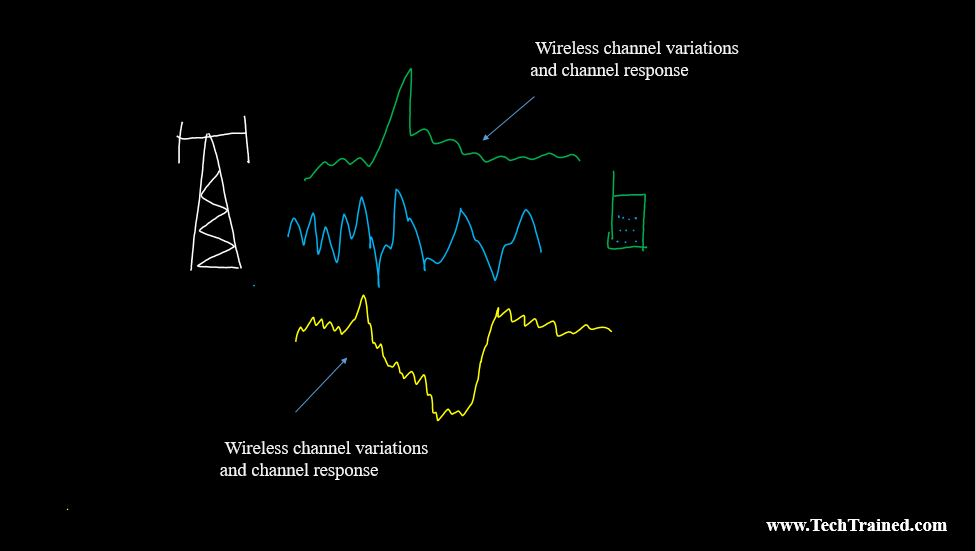For a signal to be transmitted successfully from the transmitter to the receiver, it should adapt to the variations of the wireless channel. In order to overcome the variations and channel response between the transmitter (BS) and the receiver (user). Different techniques can be used.

How to adapt the signal to these channel variations?

In order to adapt signal from the variations of the wireless channel, predefined procedures exist at Layer 2, and Layer 1 to prepare and massage the signal from getting corrupted from these variations. We are not going to list all of the ways and protection mechanisms done at these layers to protect the signal.  However , some specific ways to overcome these variations include.

• Modulation scheme
• Coding Rate etc.

POWER CONTROL vs. RATE CONTROL

When it comes to Packet switched technologies such as LTE, we are mainly concerned with Data rate, unless if you are supporting Voice Services  or other supplementary services i.e., VoLTE etc.

Let’s keep our focus on to Data Services alone for a moment. In order to provide data services, there can be two approaches to overcome channel variations.

1. Rate Control
2. Power Control

In Power Control:    Transmitted power is varied in accordance with channel quality as shown in the diagram.  Power is varied w.r.t. channel quality to provide a fixed data rate.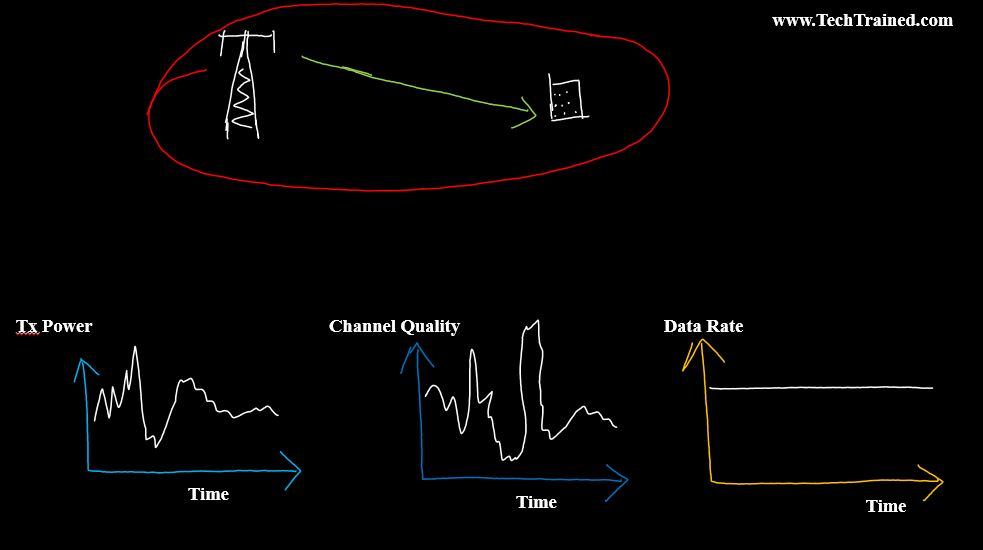In Rate Control:  Transmitted power remains fixed. However, in order to cope with variation in channel quality. Modulation and coding schemes are varied to compensate for channel variations.   In this case data rate is variable, while transmitted power remains fixed as shown in the picture below.POWER CONTROL IN LTE

Let’s focus our discussion on Power Control in LTE for now.

In LTE , Power control takes place both in Downlink and Uplink. They way it takes place in Downlink and Uplink will become evident in a little bit , as we go further down the discussion.  However, a picture is worth thousand words. Therefore the picture below should explain you on Which channels in DL and UL , power control takes place.

In case of 4G DL, rather than varying power in the Downlink, full power is distributed uniformly over the whole bandwidth. The same Power Spectral Density (PSD) is used on all DL channels. For example, PDSCH, PHICH, PDCCH etc.

How do we calculate PSD?

PSD is the power of a signal divided by Bandwidth.

PSD = Power / Bandwidth.

In case of PSD, it is normalized to one resource block.

Note: There are certain channels in DL, where power is varied accordingly. We are not going to focus on how it is done and why in this topic for the sake of discussion for now. If you have questions for power control in the Downlink, leave them in the comments below.

Instead we will focus on UPLINK POWER CONTROL, with the detailed discussion on Power control on one of the Uplink Channel as an example.

Do we Still Perform Power Control on Uplink (UL)?

As compared to Downlink. In case of Uplink in LTE, Power control is used. As the battery of the phone(UE) is power limited compared to base station power in the DL.

Uplink power control is used mainly for the following two reasons.

1. limit intracell and intercell interference
2. reduce UE power consumption

How to Perform  Power Control  for the Uplink?

Usually in Uplink. Power control is done in two ways.  One is

• Conventional Power Control
• Fractional Power control.

Conventional Power control is used in attempt to maintain a constant Signal to Interference plus Noise Ratio (SINR) at the receiver. UE increases their transmit power to fully compensate any  increase in path loss. The scheme  is shown in figure below.

Whereas for fractional Power Control scheme. It allows the received SINR to decrease as the path loss increases, i.e., the received SINR decreases as the UE moves towards cell edge. The UE transmit power at a reduced rate as the path loss increases, when compared to conventional power control, i.e, increase in path loss are only partially compensated.

Both concepts are shown beautifully in the picture below 🙂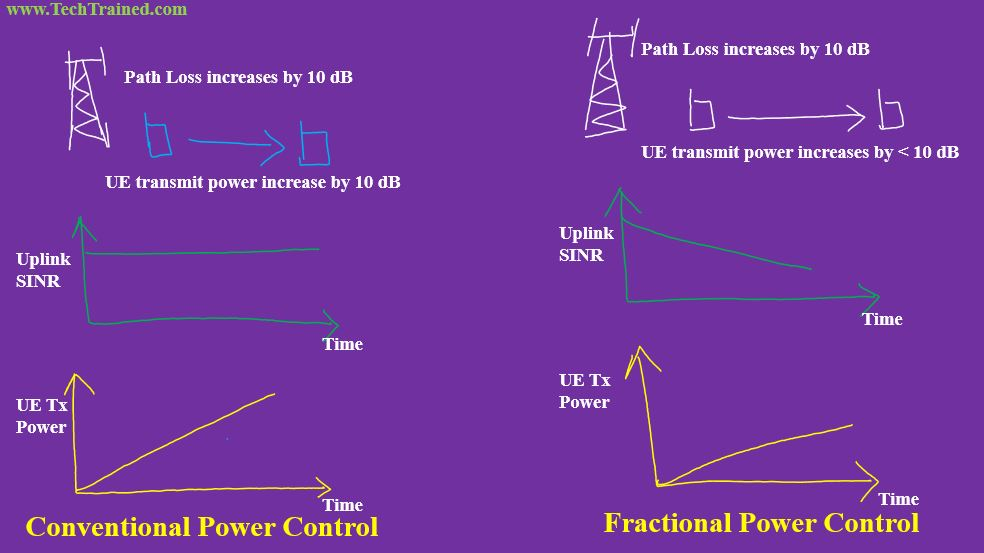What is the advantage of Fractional Power Control ?

Fractional power control scheme improves air-interface efficiency and increase average cell throughput by reducing intercell interference.

As an example and comparison among the two schemes. UE transmit power and received power spectral density as a function of path loss are shown below. (The definition of Alpha and possible values are explained in the post further down below. )UE Transmit Power and Received Power Spectral Density (PSD) as a function of path loss (Fractional Power Control )UE Transmit Power and Received Power Spectral Density (PSD) as a function of path loss ( Conventional Power Control )

Open Loop and Closed Loop Power Control?

From the perspective of power control, it is important to understand the difference between Open Loop and Close loop power control.

Open Loop Power Control Diagram.

In case of Open Loop power control. UE will start with the objective to compensate the path loss. Whether Open Loop Power control is done using Fractional or Conventional Power Control scheme. It depends on the if they enable Fractional Power control or disable it.

If fractional power scheme is used , it forms the Open Loop component of power control.

Open loop power control can maintain target PSD as received by the eNodeB. However a  disadvantage of Open Loop Power control is, it cannot compensate for issues like slow fading. Therefore we need to introduce closed loop component.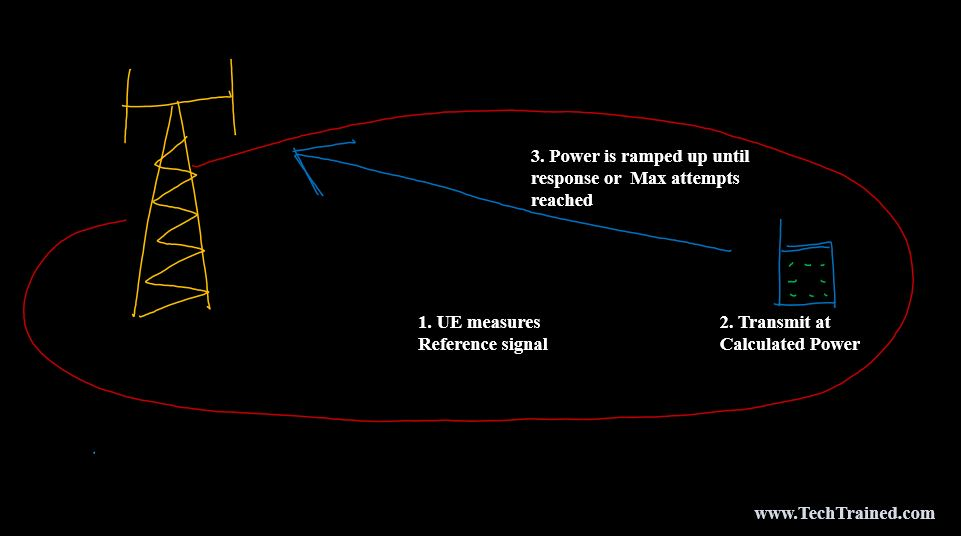Closed Loop Power Control:

Close Loop power control. In this case, the closed loop component is based on feedback given by eNodeB to the UE.  The receiver in Uplink which is eNodeB will issue Special Transmit power control command (TPC) to the UE. Based on the TPC, UE will either increase or decrease its power as instructed to compensate for the path loss.  Closed loop can compensate for issues such as slow fading.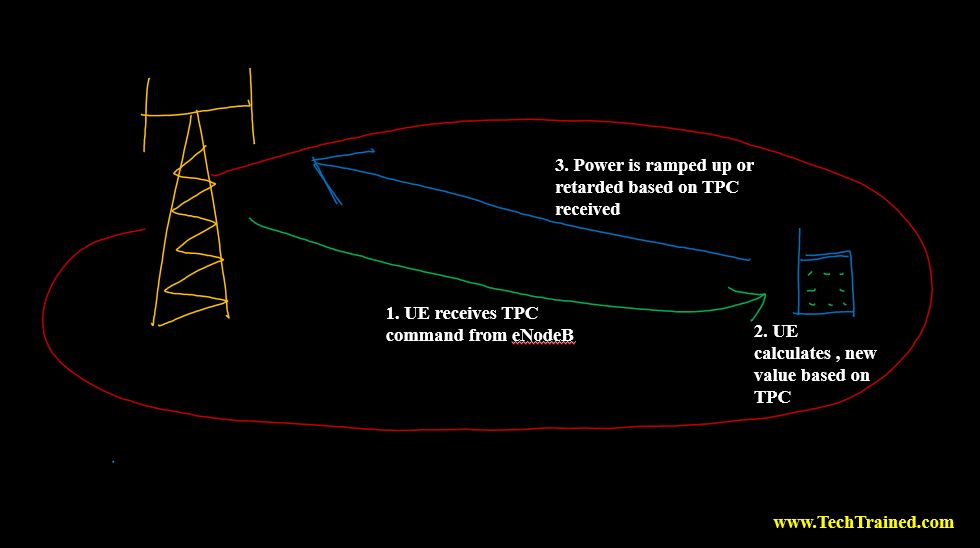Why to have Open Loop and Closed Loop Power Control ?

If you are asking , why to have two components i.e., open and close loop in the first place.

Well, a picture is wroth thousand words. Therefore take a look below.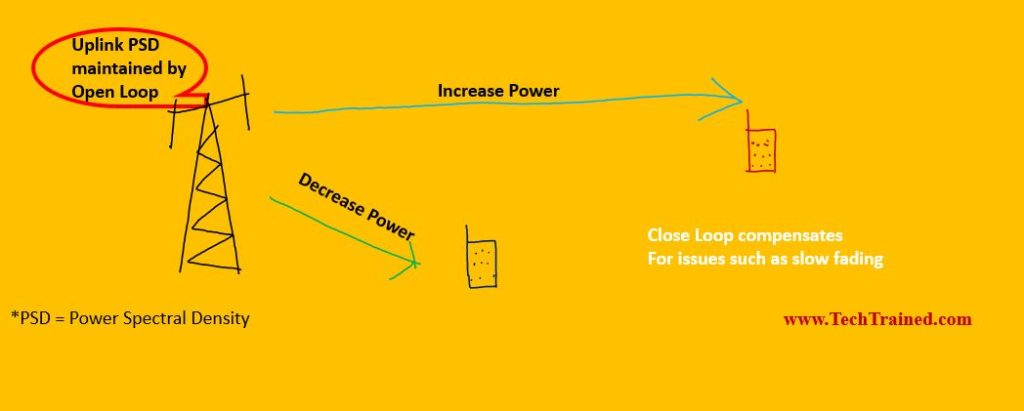Enough Background to Accelerate for Power Control on UPLINK IN LTE

If you have read down so far, Congratulations!!!! Now you are done with the necessary perquisite and background needed to understand Power Control and procedures in LTE Uplink.

Let’s dig a little deeper to perform power control on Physical Uplink Shared Channel (PUSCH)  channel.

Keep in mind the function of this PUSCH channel is to

• Carry Data Traffic in the Uplink
• It can also carry signaling traffic in the Uplink, only when signaling and data are being transmitted at the same time instant in the Uplink.

Side Note : For those who don’t want to go through the gory details(for your mind) of the Power control components and parameters for PUSCH. Do yourself a favor, scroll all the way down on this article and read the Quick Summary instead (4.4 mins read) .

What factors influence Power Control in Uplink on PUSCH

Now you know, what is the functionality and usage of PUSCH channel.

What factors are going to affect power control on the Uplink for PUSCH.Look at the picture above.  You know which channel we are talking about here. We are talking about PUSCH only. PUSCH = Channel which carries data traffic in the uplink and can also carry control signaling when required/needed.

For this PUSCH channel. Power control will depend upon mainly on the following factors  (though a lot more shown in picture ) .

• Number of Resources
• MCS
• Path Loss
• UE Max Power

The detailed number of parameters are listed below.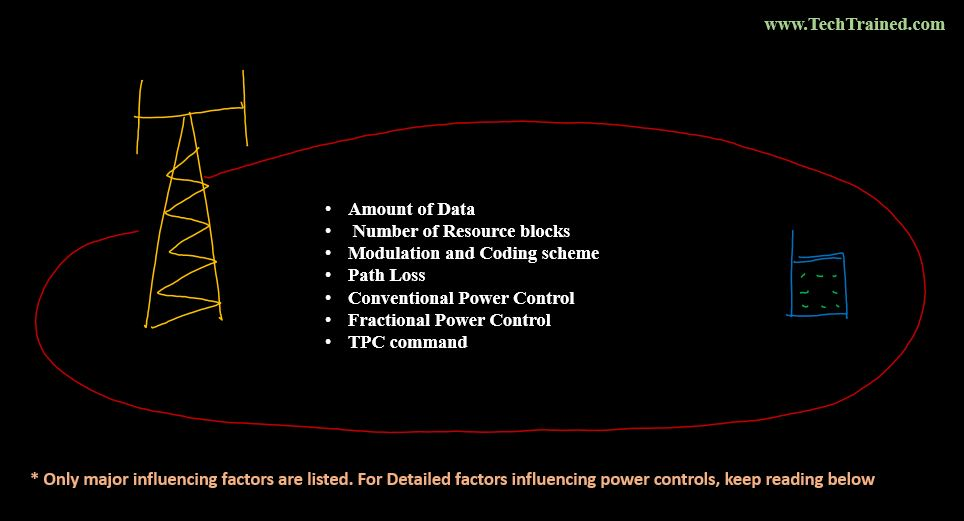If you feel confused to see a number parameters listed above. Don’t be.

Let’s deal with these parameters on one by one, to keep our attention span sane.

UE MAX POWER

As we know based on UE class category. It cannot transmit more than its maximum UE power which is commonly 23 dBm for most LTE UEs in the Uplink.

The objective of the eNOdeB is that UE should transmit only enough power in the uplink in a range which is the minimum required amount. Neither more, nor less than that.

Transmitting more or less than the required amount causes trouble.

UE Power Max: 23 dBm

Accordingly, there are limits and thresholds specified for transmission in the uplink to keep UE uplink power in the desired range.  UE power is specified as Pcmax

PCMAX_L ≤ PCMAX ≤ PCMAX_H

Where,

PCMAX_L = lower end of the maximum power UE is allowed to transmit

PCMAX_H       = higher end of the maximum power UE is allowed to transmit.

As an example , if  23 dBm = PCMAX_H

and 21 dBm = PCMAX_L

in this case UE would be permitted to define its maximum output power using a value between 23 and 21 dBm.

(Note: If you have specific questions on P_CMAX_L and P_CMAX_H . Put in the comments below or send an email and we can continue the discussion)

Even though if we think intuitively, Higher uplink transmission Power can solve the Uplink throughput and accessibility and retainability issues problems related to Performance and Optimization.

However, the downside is,  it will not only drain UE battery,  plus it will result increase in inter and intra cell interference as well.

Therefore, you need to be careful with the parameters, and different factors affecting power control. By understanding the big picture and factors involved, you can always decide and optimum value.

MODELING THE POWER CONTROL on PUSCH

Now let’s come back to the part where we will model the power control equation for PUSCH channel on LTE in Uplink.

We know that we need to model the power in the uplink to an optimal value between lowest and highest value.

PUSCH Power Conrol

We know the maximum value is

Pcmax = Pupper

And the minimum value will be equal to the one needed by the UE based on the calculations and including the factors affecting power control.

With this in mind, for a given subframe ‘i’ the power transmitted for PUSCH can be a minimum of the two.

PUSCH Transmit Power (i) = min { PUPPER (i), PCALCULATED(i)}

Time to Break down, Pcalculated:

Pcalculated is the sum of Open Loop and Close Loop.

Pcalculated (i) = P calculated_open_loop (i) + P calculated_closed_loop(i)

Open Loop for PUSCH:

Open loop is dependent upon the factors which affect power control. Let’s list the factors which only affect Open Loop Power control for PUSCH. Mentioned in the diagram below.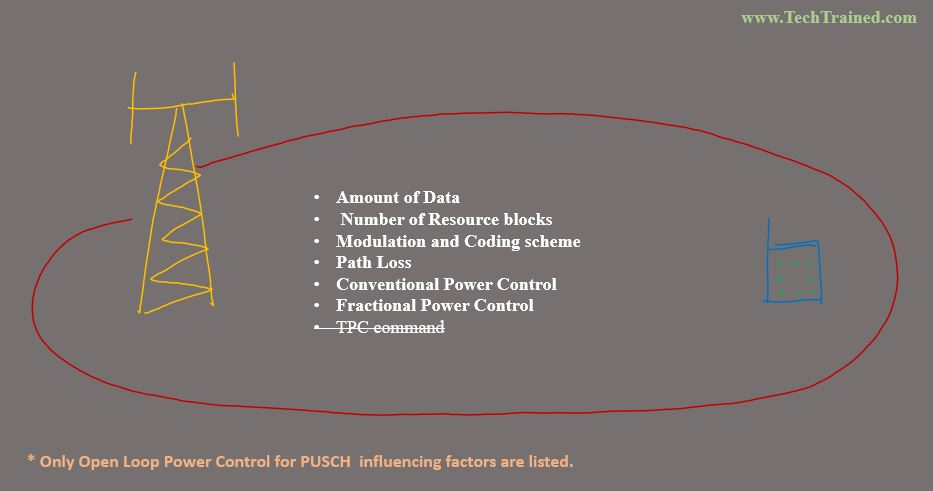Therefore, arranging the open loop power control factors into an equation , to consolidate its effect.

P calculated_open_loop = 10*LOG (# of Resource Blocks) + Power needed at eNodeB +  (Path_Loss* Factor to Enable or Disable Fractional Power Control )  + MCS

Let’s break down the factors discussed in the equation  above into reasonable expressions for Power Control on PUSCH.

# of Resource Blocks for PUSCH = MPUSCH

MPUSCH is the PUSCH bandwidth during subframe ‘i’ expressed in terms of Resource Blocks. This variable is used to increase the UE transmit power for larger resource block allocations.

The UE transmit power is increased in direct proportion to the number of allocated Resource Blocks.

In other words, the transmit power per Resource Block remains constant if other factors remain fixed. This is also referred as maintaining a constant power spectral density

Path Loss = PL

Path loss is the downlink path loss calculated by the UE as a combination of RSRP measurements and knowledge of the reference signal transmit power

PL = Reference Signal Transmit Power – RSRP measurements.

The Reference signal transmits power is broadcast within SIB 2 and can also be signaled with an RRC Connection Reconfiguration message. Its value range from -60 to 50 dBm.

Power needed at eNodeB = Po_PUSCH

Po_PUSCH represents the eNodeB received power per Resource Block assuming a path loss of 0 dB.

The received power per Resource Block is maintained as the path loss increases when using conventional power control alone.

The received power per Resource Block is decreased as the path loss increases when using fractional power control.  (Details are below).

Factor to enable or disable Fractional Power Control   =   α

Alpha (α ) is used to configure the use of fractional power control. This is the same variable as that used by the eNodeB when calculating Po_PUSCH.

A value of 1 disables fractional power control.

Alpha can have a range of values from

• 0,
• 0.4,
• 0.5,
• 0.6,
• 0.7,
• 0.8,
• 0.9,
• 1

Modulation and Coding scheme = ∆TF

It increases the UE transmit power when transferring a large number of bits per Resource Element. This links the UE transmit power to the Modulation and Coding Scheme (MCS). The number of bits per Resource Element is high when using 64 QAM and a large transport block size. The number of bits per Resource Element is low when using QPSK and a small transport block size. Increasing the UE transmit power helps to achieve the SINR requirements associated with higher order modulation schemes and high coding rates.

Now combining all the expressions listed above into the OPEN LOOP Equation.

PCALCULATED_OPEN_LOOP = 10×LOG(MPUSCH) + PO_PUSCH + [PL × ɑ] + ∆TF

As for power control, if we want to include the factor to include power control at subframe (basis)  level. Let’s call the subframe ‘i’.

The above equation will become.

PCALCULATED_OPEN_LOOP(i) = 10×LOG(MPUSCH(i)) + PO_PUSCH(i) + [PL × ɑ(i)] + ∆TF(i)

CLOSED LOOP PORTION

P calculated_closed_loop = f(i)

The closed loop component depends on eNodeB providing feedback to the UE in the form of Transmit Power Control (TPC) commands.

The close loop portion of the power control depends on the following factors.For those who have firm grip on LTE air interface and control channel structure scheme of LTE, they will agree that TPC commands are signaled to the UE within following Downlink Control Information (DCI) formats

• DCI forma 0
• DCI format 3
• DCI format 3A
• DCI format 4

Interpretation and application of TPC commands depends on if either accumulation mode is enabled or not. UE can be instructed about setting it up or not in RRC messages.

When TPC Accumulation Mode is Enabled:

If it is enabled then, closed loop portion is replaced as follows below.

f(i) = f(i-1) + δPUSCH (i – KPUSCH)

δPUSCH (i – KPUSCH) is the TPC command received during subframe ‘i-Kpusch’.

KPUSCH = 4 for FDD

For TDD. Kpusch is dependent upon the UL-DL subframe configuration and subframe number.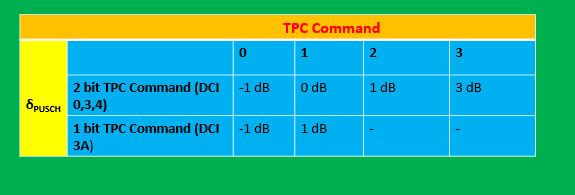When TPC Accumulation Mode is Disabled:

In case when TPC is disabled, then feedback close loop equation becomes.

f(i) = + δPUSCH (i – KPUSCH)

This mode only uses TPC commands sent on DCI formats 0 and 4.

It has more range of commands compared to accumulation mode, to compensate for not being able to sum multiple commands over time.What is the ROLE OF Po_PUSCH?

Let’s repeat the definition of this Po_PUSCH once again.

“Po_PUSCH represents the eNodeB received power per Resource Block assuming a path loss of 0 dB.

The received power per Resource Block is maintained as the path loss increases when using conventional power control alone.

The received power per Resource Block is decreased as the path loss increases when using fractional power control.”

eNodeB takes into account the following factor to receive a target power from UE:

• Target SINR
• Interference and noise per Resource Block at the eNB
• UE maximum power to transmit
• Number of allocated resource blocks
• Factor to enable / disable Fractional Power Control.

Keeping above factors in mind. The way to calculate Po_PUSCH is:

PO_PUSCH = ɑ × (SINR + IN) + (1 – ɑ) × (PCMAX – 10 × LOG(MPUSCH))

Po_PUSCH Use Cases

For Alpha (ɑ) = 1:

Value of Po_PUSCH is smallest

For Alpha (ɑ)  < 1 :

Value of Po_PUSCH increases as value of Alpha. UE transmit power only partially compensates the path loss when fractional power control is enabled.

For Alpha (ɑ) = 0:

Value of Po_PUSCH is maximum when alpha = 0. Corresponds to maximum transmit capability of the UE, irrespective of the path loss.

Po_PUSCH Components:

Po_PUSCH depends on two factors as listed in the equation above.

• Po_NOMINAL_PUSCH
• Po_UE_PUSCH

Therefore, Po_PUSCH is signaled to the UE as a combination of the above two factors. In equation form, it can be written as shown below.

PO_PUSCH(i) = PO_NOMINAL_PUSCH(i) + PO_UE_PUSCH(i)

Nominal: Specifies the cell specific factor / component. Its value can be anywhere between – 126 to 24 dBm.

Po_PUSCH (0) It can be signaled during RRC Connection Setup, RRC Connection Reconfiguration and RRC Connection Re-establishment messages.

Po_PUSCH(1): It is broadcast in SIB2 or can be signaled within RRC configuration message.

Po_PUSCH (2): We will not discuss it in this post

Po_UE_PUSCH:  represents the UE specific component.

Performance Engineers Note:  So far we have discussed about the ins and out of Power control on PUSCH and the factors affecting it. Configuring and changing any of the above mentioned parameters, will dramatically change any of the following:

•  power control on PUSCH,
• UE battery consumption,
• interference,
• Performance KPIs such Accessibility, Retainability etc.

In practice, usually the value of P0_NOMINAL is the only one available parameter most Telecom vendors offer to operators in order to change or configure uplink power control parameters in Uplink for PUSCH.

Increasing the value of P0_NOMINAL   avoid interference. It improves uplink throughput and accessibility but will result in increase of inter cell interference. Therefore, care should be taken while keeping an eye on network performance when changing this value.

QUICK SUMMARY    (Approx. 4.4 Minutes Read )

Having said enough about PUSCH power control. Let’s go through the quick summary now and

review what we have learned so far.

Ready let’s jump right in  !!!

In order to perform power control in the Uplink, keep in mind there are two portions.

• Open Loop Power control
• Closed Loop Power Control.

What factors Uplink Power for PUSCH depends on?

Thinking about, on what factors power control depends upon?

To make your life easier. Power control in the uplink on PUSCH channel depends on the following factors shown below in the picture.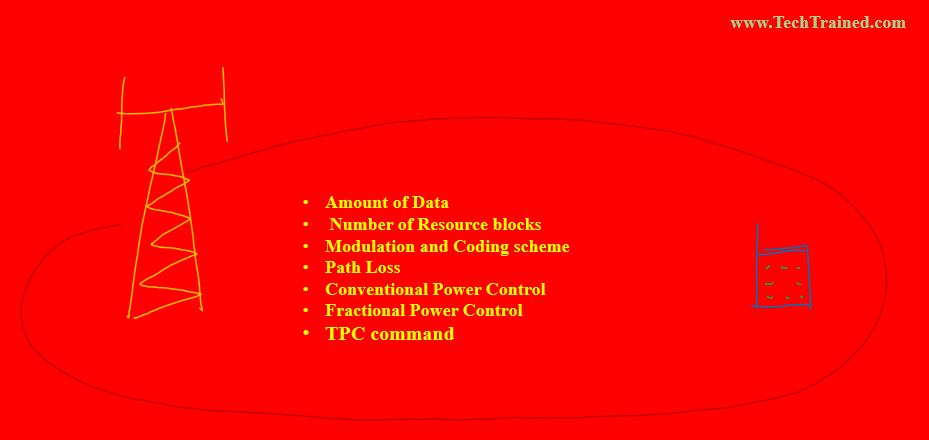(In order to understand why power control in Uplink for PUSCH depends on the factors listed below in the picture. Read the article from the start once again 🙂 )

Ideally, uplink power needs to be 0nly minimally required as needed to avoid interference and decrease UE batter consumption. Therefore, Power on PUSCH is computed as minimum

among the UE max power and power computed taking factors into account

Power on Uplink PUSCH =  Min (UE max power ,  Power computed through dependency factors listed above on red canvas )

Writing this mathematically , it becomes.

PPUSCH = min { Pcmax , 10×LOG(MPUSCH(i)) + PO_PUSCH(i) + [PL × ɑ(i)] + ∆TF(i) + f(i)}

Where,

1. PCMAX = configured UE transmitted max power defined in TS 36.101
2. Po_PUSCH = Target Power Spectral Density
3. MPUSCH = Number of assigned resource blocks
4. PL = Estimated Downlink Path Loss
5. α = Factor to enable or disable Fractional Power Control (also termed as Cell Specific factor)
6. f(i) = Closed loop component of Power control (TPC commands)
7. TF    = Modulation and coding scheme (transport format-depending compensation)

Open Loop Portion of the above Equation is Shown as:

POPEN_LOOP_PUSCH = min { Pcmax , 10×LOG(MPUSCH(i)) + PO_PUSCH(i) + [PL × ɑ(i)] + ∆TF(i)

Closed Loop Portion of the above Equation is Shown as:

PCLOSED_LOOP_PUSCH = f(i)

MAIN OPERATION

First UE will set up the operating point of Target power to be received by the eNodeB in the uplink, using Open Loop Power control.

In order to compensate for any effects such as slow fading etc. Close Loop portions kicks in , once eNodeB gives feedback using TPC commands.

Taking that feedback into account, UE will increase or decrease its power in the uplink.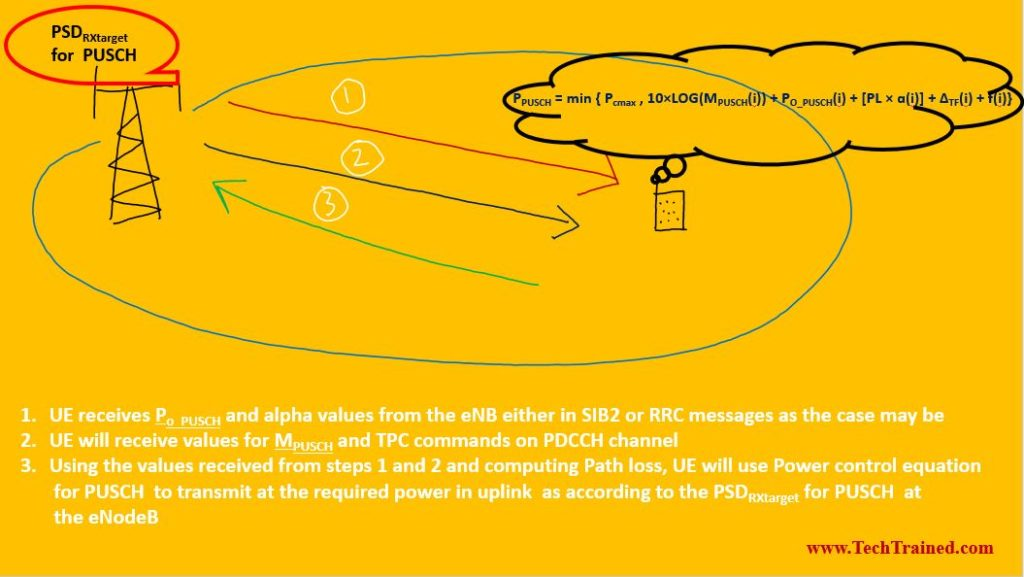The procedure will run, based on the TPC commands being received. In case of LTE for uplink. Power control command is received every 5th sub frame, which means power control takes place 200 times a second, which is much lesser than previous technologies, where power control can take place up to 1500 times a second.

Alright good deal.  This quick summary , gives you a high level idea on :

• How power control takes place on PUSCH channel.
• What factors are involved in power control.
• What can be configured to improve performance and/or optimize the network.

If you have read all of the details above. Then , do the following.

2.  If you found it helpful reading this post. Do your friends and colleagues a favor by sharing it with them.
3. If you would like to see more detailed posts like this right in your inbox, so that you never lose them.  Sign Up Below.

Sources:

TS 36.213, TS 36.101, LTE in Bullets, TS 36.331

•Sanil Talauliker says:

What does the capital I mean in this formula f(i) = + δPUSCH (I – KPUSCH)?

•Azar says:

Sanil, that capital ‘I’ is a typo. It should be read as ‘i’. It represents the sub-frame number in which TPC command is received. It has been corrected.

•Zikovich says:

Thanks a lot for the useful information.
I suggest to have some reflection to PHR and carrier aggregation power control.

Thanks again Azar.

•chris weglinski says:

How would the # of Simultaneously connected UE’s be impacted in an Indoor DAS Systems where the RF Conditioning tray is using Added Attenuation to “negate” the Cascaded Noise of the Active and Passive gear “homed” to any Single DAS Sector fed by an eNb running 2X2 LTE with 15MHZ downlink and 15MHZ Uplink?

•Kaushik K S says:

I wanted to know if Power Control is implemented in the Downlink of 4G LTE. If yes, can you please explain it?

•Azar says:

Kaushik, I will compile a write up post for it and send it your way, as schedule permits

•james Val says:

So what if I apply blanking to the Resource block, what would be the power for the Un-blanked RB and the blank RB separately?

•Parteek Garg says:

Hi Azhar,
I must say this is really very good explanation and easy to understand.
Well written and explained.
I have one query –
So we have Open loop SINR adjustment in LTE, what you say for LTE TDD? How will it work keeping in mind different Frame structure.
We can set Target BLER and SINR to mitigate poor RF conditions so that our varying signal does not makes much difference in performance. Keeping in mind HARQ process comparatively complex in TDD as compare to FDD, How will it work in case TDD if we want to set Target BLER and SINR.

•Azar says:

Thank you for the kind words Prateek

•Azar says:

In TDD-LTE, the delay between transmission and the HARQ ACK/NACK depends on both the TDD configuration and the subframe in which the data was transmitted.
The delay tables have been fixed by the standard for different implementations of HARQ in TDD and the delay is expected to follow the description given for the respective cases.

Once you have a given scenario of TDD radio frame configuration and subframe specified. Based on that specific case and following table you can specify target BLER and SINR.

Thank you for the question